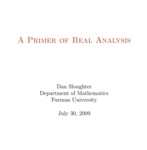## Open Textbooks#### Title

A Primer of Real Analysis

#### Contributors

Dan Sloughter - Author

#### Description

This is a short introduction to the fundamentals of real analysis. Although the prerequisites are few/ I have written the text assuming the reader has the level of mathematical maturity of one who has completed the standard sequence of calculus courses/ has had some exposure to the ideas of mathematical proof (including induction)/ and has an acquaintance with such basic ideas as equivalence relations and the elementary algebraic properties of the integers.

#### Subject 1

Mathematics - Applied

#### Subject 2

Mathematics - Applied

Dan Sloughter

#### Resources

Open Textbook Library